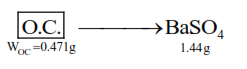# In the sulphur estimation

Question:

In the sulphur estimation, $0.471 \mathrm{~g}$ of an organic compound gave $1.44 \mathrm{~g}$ of barium sulphate. The percentage of sulphur in the compound is ____________. $\%$

(Nearest integer)

(Atomic Mass of $\mathrm{Ba}=137 \mathrm{u}$ )

Solution:

Molecular mass of $\mathrm{BaSO}_{4}=233 \mathrm{~g}$.

$\because 233 \mathrm{BaSO}_{4}$ contain $\rightarrow 32 \mathrm{~g}$ sulphur

\therefore 1.44 \mathrm{~g} \mathrm{BaSO}_{4} \text { contain } \rightarrow \frac{32}{233} \times 1.44 \mathrm{~g} \text { sulphur }

given : $0.471 \mathrm{~g}$ of organic compound

$\%$ of $\mathrm{S}=\frac{32 \times 1.44}{233 \times 0.471} \times 100=41.98 \% \approx 42 \%$

OR$\Rightarrow \mathrm{n}_{\mathrm{s}}=\mathrm{n}_{\mathrm{BaSO}_{4}}=\frac{1.44}{233}$

$\Rightarrow \mathrm{w}_{\mathrm{s}}=\frac{1.44}{233} \times 32 \mathrm{~g}$

therefore $\% \mathrm{~S}=\frac{\mathrm{W}_{\mathrm{s}}}{\mathrm{W}_{\text {O.C. }}} \times 100=\frac{1.44 \times 32}{233 \times 0.471} \times 100$

$=\frac{46.08}{109.743} \times 100=41.98 \simeq 42$